# (Solved): could you answer those textbook questions based on my lab please! HCl: clear, 98.5mL, transparen ...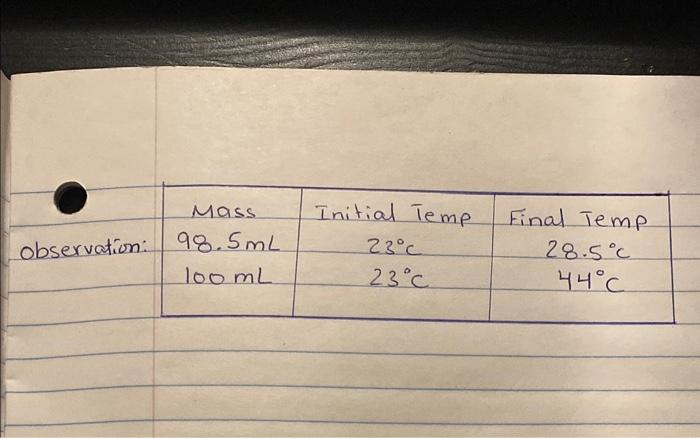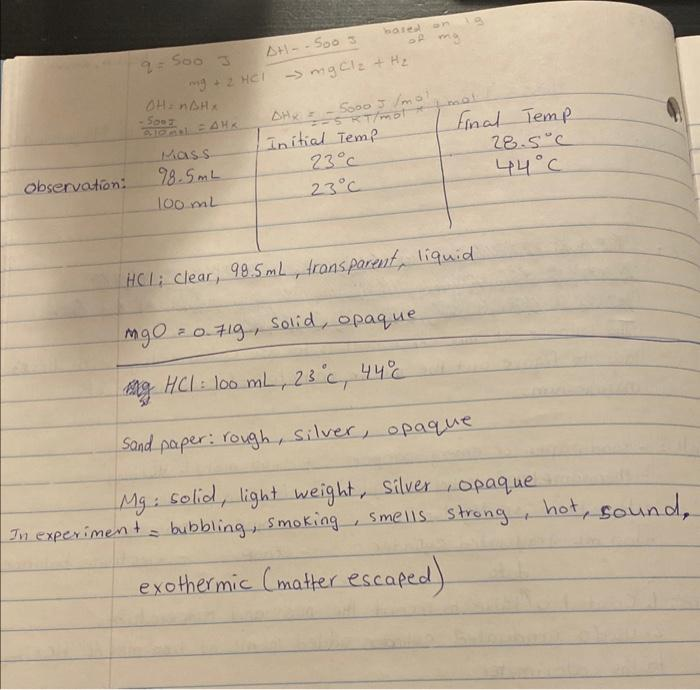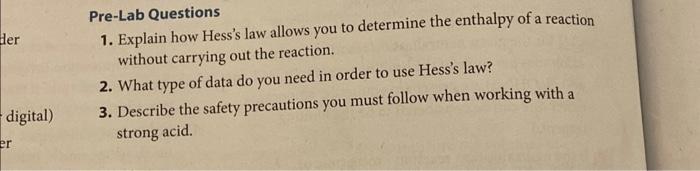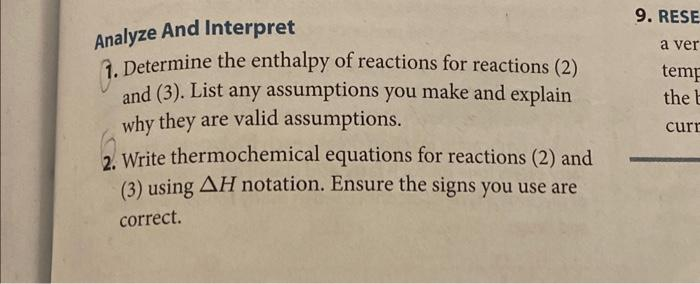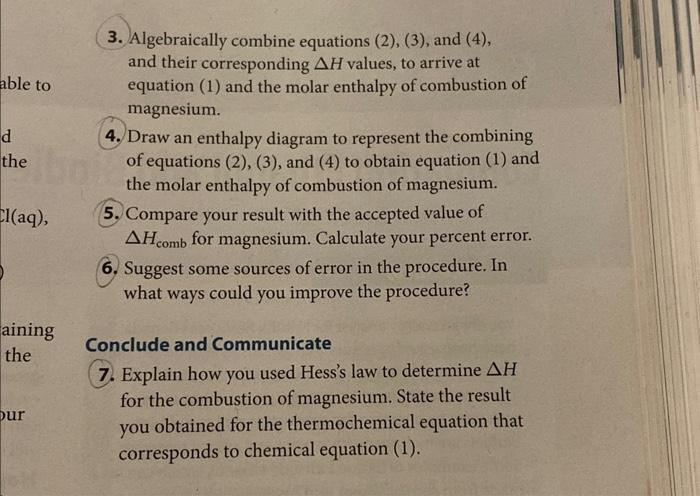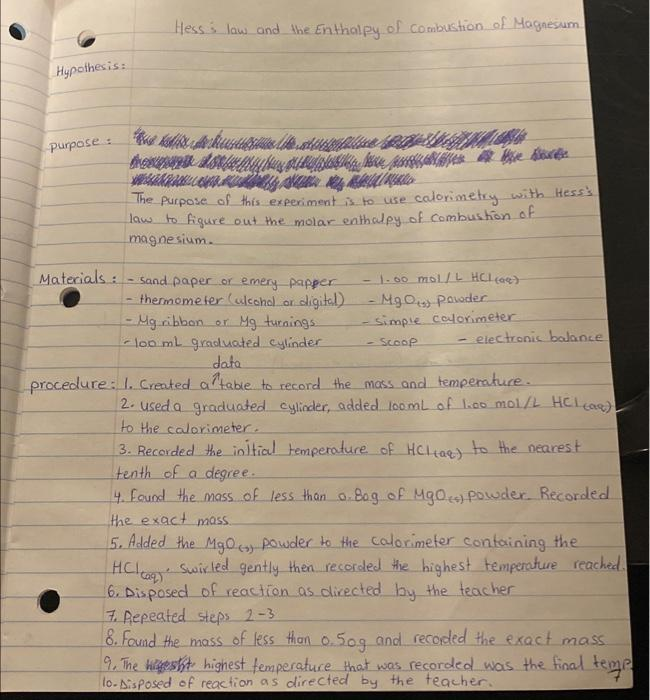HCl: clear, , transparent, liquid Sand paper: rough, silver, opaque : solid, light weight, silver, opaque In experiment = bubbling, smoking, smells strong, hot, sound, exothermic (matter escaped) Pre-Lab Questions 1. Explain how Hess's law allows you to determine the enthalpy of a reaction without carrying out the reaction. 2. What type of data do you need in order to use Hess's law? 3. Describe the safety precautions you must follow when working with a strong acid. Analyze And Interpret 1. Determine the enthalpy of reactions for reactions (2) and (3). List any assumptions you make and explain why they are valid assumptions. 2. Write thermochemical equations for reactions (2) and (3) using notation. Ensure the signs you use are correct. 3. Algebraically combine equations (2), (3), and (4), and their corresponding values, to arrive at equation (1) and the molar enthalpy of combustion of magnesium. 4. Draw an enthalpy diagram to represent the combining of equations (2), (3), and (4) to obtain equation (1) and the molar enthalpy of combustion of magnesium. 5. Compare your result with the accepted value of for magnesium. Calculate your percent error. 6. Suggest some sources of error in the procedure. In what ways could you improve the procedure? Conclude and Communicate 7. Explain how you used Hess's law to determine for the combustion of magnesium. State the result you obtained for the thermochemical equation that corresponds to chemical equation (1). Hess: law and the Enthalpy of Combustion of Magnesum Hypothesis: purpose : The purpose of this experiment is to use calorimetry with Hess's law to figure out the molar enthalpy of combustion of magnesium. date procedure: 1. Created a table to record the mass and temperature. 2. used a graduated cylioder, added of (aq) to the calorimeter. 3. Recorded the initial temperature of to the nearest tenth of a degree. 4. Found the mass of less than of powder. Recorded the exact mass 5. Added the powder to the calorimeter containing the swirled gently then recorded the highest temperature reached 6. Disposed of reaction as directed by the teacher 7. Repeated steps 8. Found the mass of less than and recooled the exact mass 9. The hilgesht highest femperature that was recorded was the final tem 10. Disposed of reaction as directed by the teacher.

We have an Answer from Expert